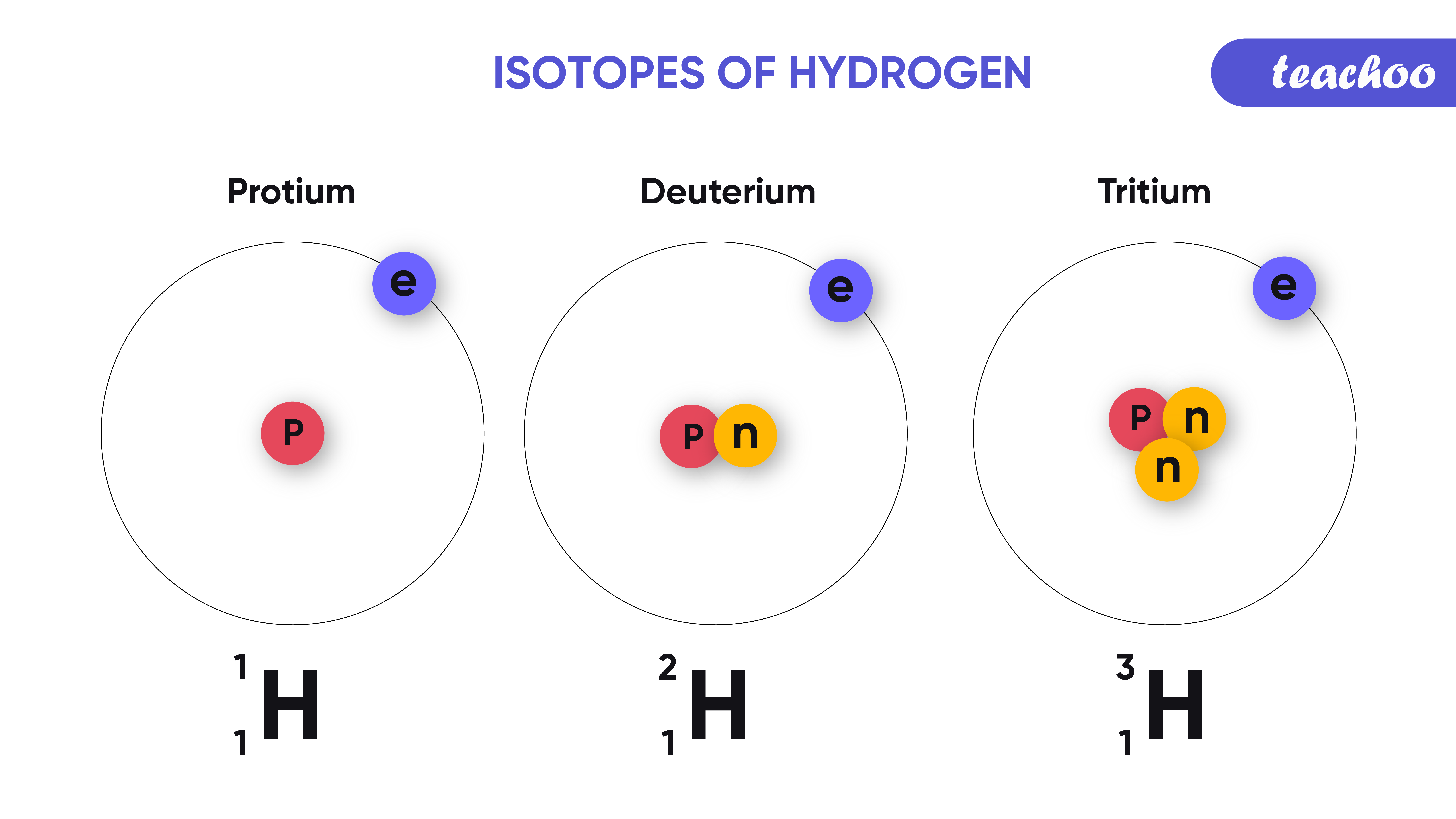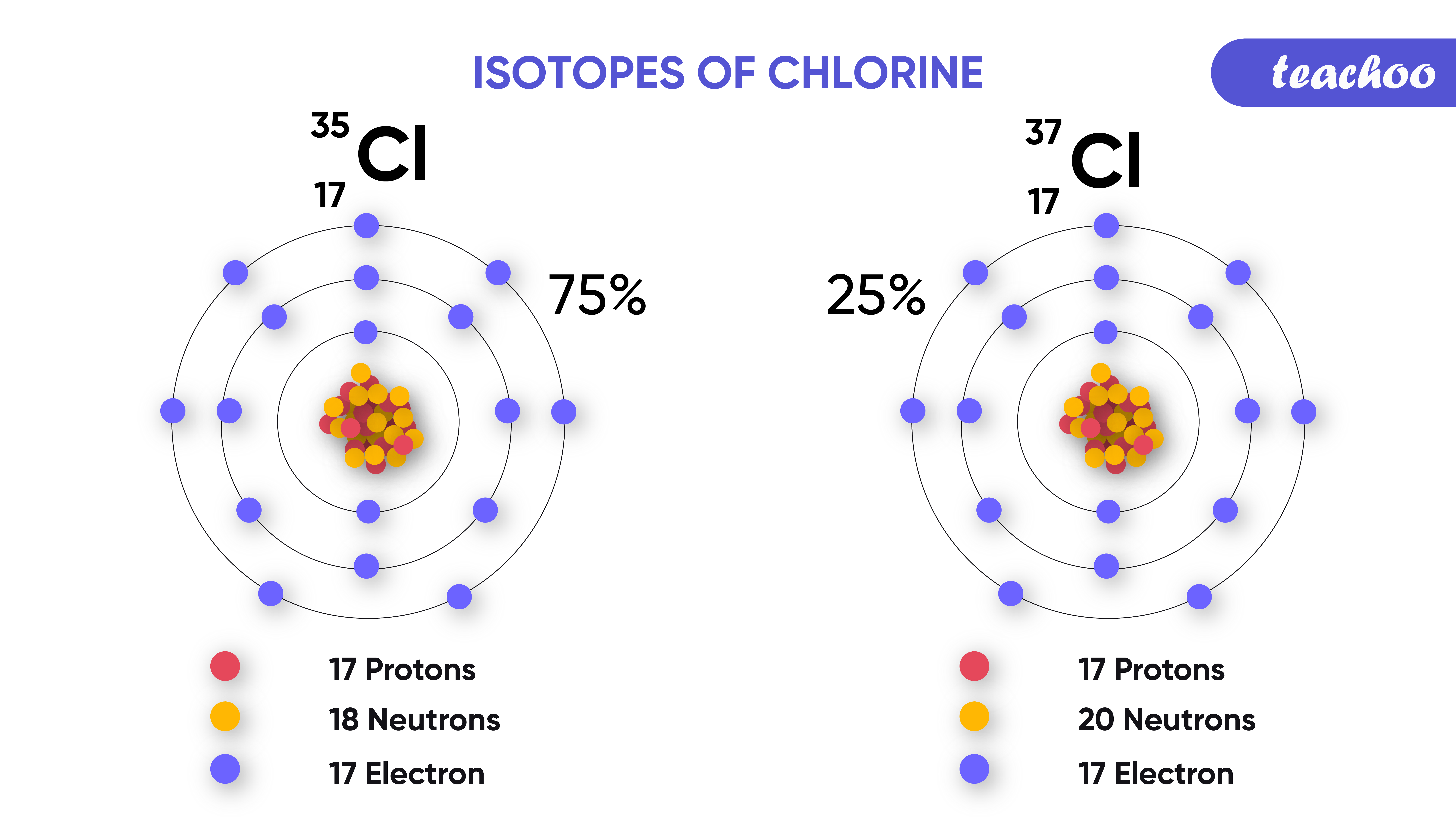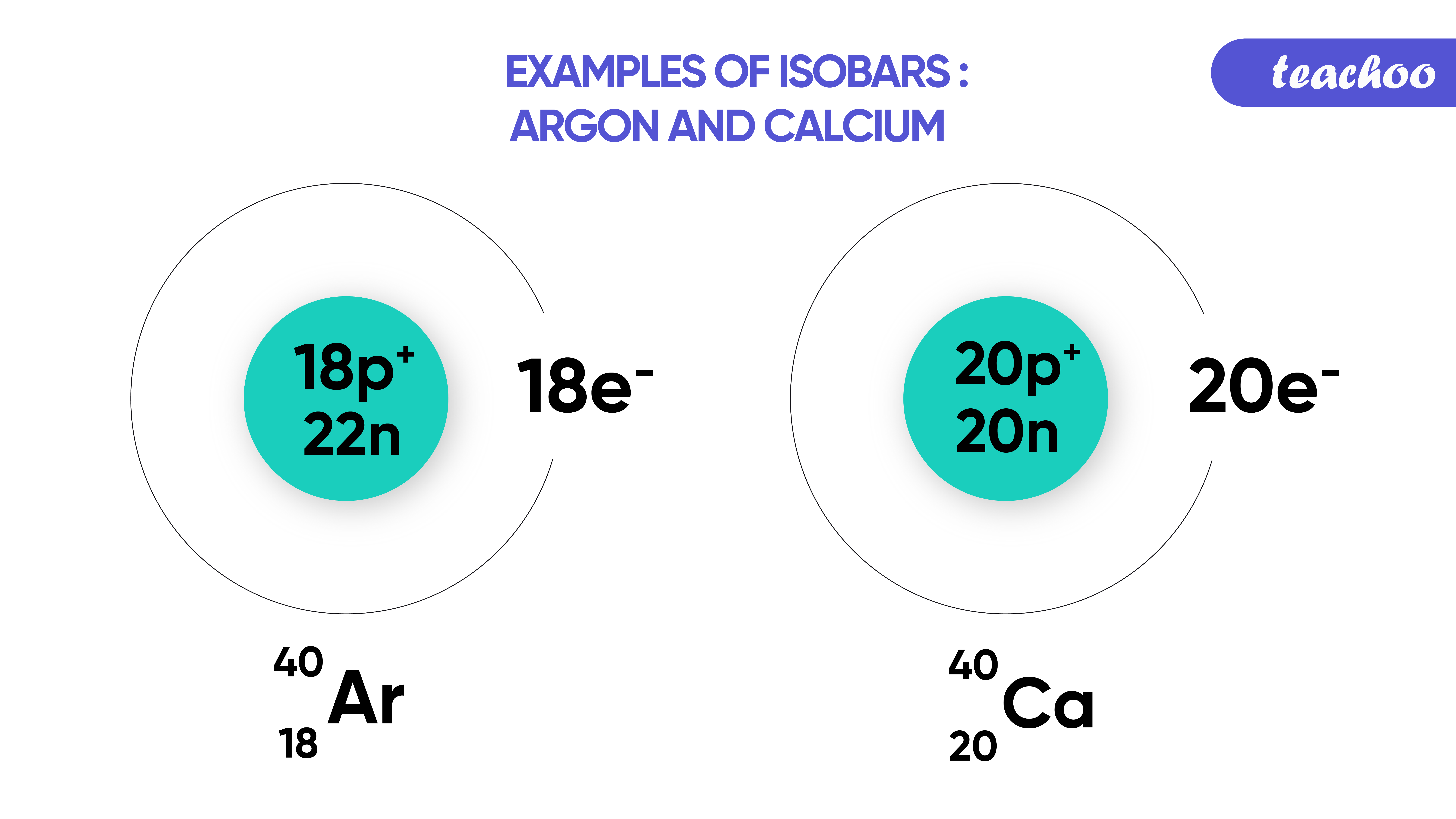Concepts

Class 9
Chapter 4 Class 9 - Structure of Atom

## What are isotopes?

Atoms of same element which have different mass number but same atomic number

## Example - 1

• Hydrogen Element has 3 isotopes:-
1. Protium
2. Deuterium
3. Tritium## Difference between Different Isotopes of Hydrogen

 Protium Deuterium Tritium It has an atomic number = 1 and mass number = 1. It has atomic number = 1 and mass number = 2. It has atomic number = 1 and mass number = 3. It has only 1 proton and 0 neutron It has only 1 proton and 1 neutron. It has only 1 proton and 2 neutrons. Protium for hydrogen is denoted as Deuterium for hydrogen is denoted as Tritium for hydrogen is denoted as

## Example - 2

• Chlorine Element has 2 isotopes:-
1. Chlorine - 35
2. Chlorine - 37

## Difference between Different Isotopes of Chlorine

 Chlorine-35 Chlorine-37 It has an atomic number 17 and mass number 35. It has an atomic number 17 and mass number 37. Nuclear composition of Cl-35 isotope is = 17 protons + 18 neutrons. Nuclear composition of Cl-37 isotope is = 17 protons + 20 neutrons. Chlorine-35 is denoted as Chlorine-37 is denoted as## How is Mass of Isotope Calculated?

• It is the average of mass of both isotopes.
• The mass of isotopes depends on how much of which isotope exists in nature .

Example

• Chlorine Element has 2 isotopes:-

Chlorine-35 →  It has a mass of 35 u, 75% of chlorine exists in the form of this isotope of chlorine.

Chlorine-37 →  It has a mass of 37 u, 25% of chlorine exists in the form of this isotope of chlorine

• The relative mass of chlorine atom can be calculated as follows:-

Average Mass of Chlorine = (35 + 37)/2

=  35.5 u

Mass of chlorine = [(75/100) x 37] + [(25/100) x 35]

= [(3 x 37)/4] + [(1x35)/4]

= 35.5 u

Hence, a general formula can be derived as follows: -

Average mass of an isotope = ( % existence of the isotope 1 x mass of isotope 1) +

( % existence of the isotope 2 x mass of isotope 2) +

( % existence of the isotope 3 x mass of isotope 3) and so on...

## Properties of isotopes

• They have same number of Protons and hence same atomic number
• Chemical Properties of Different Isotopes are similar
• However Physical Properties of Different isotopes are different

## Applications/Uses of isotopes

1. Isotope of Uranium  (Uranium 235)
It is used as fuel in Nuclear Reactors

2. Isotope of Cobalt (Cobalt-60)
It is used for treatment of cancer

3. Isotope of Iodine (Iodine 131)
It is used for treatment of goitre

Examples -

In text Question - Page 53 Q1, Q2

NCERT Back Exercise - Q8(iii), Q10, Q11, Q13, Q14(iv), Q16

## What are Isobars?

Atoms of Different elements have the same mass number , but different atomic numbers are called Isobars.## Example

• Calcium atoms have atomic number 20 and mass number 40.
• Argon has atomic number 18 and mass number 40.
• Since both have the same mass number.
• Calcium and Argon are Isobars.

Note - Argon has 18 protons + 22 neutrons = Atomic mass = 40

Calcium has 20 protons + 20 neutrons = Atomic mass = 40

## Difference between Isotopes and Isobars

 Isotopes Isobars Isotopes are atoms of the same element. Isobars are atoms of different elements. They have the same atomic number but different mass number. They have different atomic numbers but same mass numbers. Number of protons and electrons in isotopes are the same . Number of protons and electrons in isobars is different . They have the same chemical properties. They have different chemical properties. Example: Isotopes of Hydrogen - Protium, Deuterium, Tritium Example: Isobars - Carbon and Argon.

Examples -

In text Question -

NCERT Back Exercise - Q8(iv)

Introducing your new favourite teacher - Teachoo Black, at only ₹83 per month